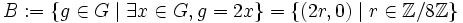# Characteristic not implies fully invariant in finite abelian group

Jump to: navigation, search
This article gives the statement and possibly, proof, of a non-implication relation between two subgroup properties, when the big group is a finite abelian group. That is, it states that in a finite abelian group, every subgroup satisfying the first subgroup property (i.e., characteristic subgroup) need not satisfy the second subgroup property (i.e., fully invariant subgroup)
View all subgroup property non-implications | View all subgroup property implications

## Statement

In a finite abelian group, a characteristic subgroup need not be a fully invariant subgroup.

## Proof

Let$G$ be the direct sum of the infinite cyclic group and the cyclic group of order two:$G := \mathbb{Z}/8\mathbb{Z} \oplus \mathbb{Z}/2\mathbb{Z}$.

Let$H$ be the cyclic subgroup of$G$ generated by$(2,1)$.

### The subgroup is characteristic

Set:$A := \{g \in G \mid \exists x \in G, 4x = 2g \} = \{ (2r,s) \mid r \in \mathbb{Z}/8\mathbb{Z}, s \in \mathbb{Z}/2\mathbb{Z} \}$

and$B := \{g \in G \mid \exists x \in G, g = 2x \} = \{ (2r,0) \mid r \in \mathbb{Z}/8\mathbb{Z} \}$.

and:$C := \{ g \in G \mid \exists x \in G, 8x = 2g \} = \{ (4r,s) \mid r \in \mathbb{Z}/8\mathbb{Z}, s \in \mathbb{Z}/2\mathbb{Z} \}$.

Thus, we have:$D := A \setminus (B \cup C) = \{ (2r,1) \mid r \in \mathbb{Z}/8\mathbb{Z}, r \notin 2\mathbb{Z}/8\mathbb{Z} \}$.

Clearly, any automorphism of$G$ sends$A$ to itself, sends$B$ to itself, and sends$C$ to itself. Thus, any automorphism of$G$ sends$A \setminus (B \cup C)$ to itself. Thus, any automorphism of$G$ sends$D$ to itself. Note that$D$ comprises precisely those elements of$H$ that have the second coordinate equal to$1$: in particular,$(2,1) \in D \subseteq H$, so the subgroup generated by$D$ equals$H$. Thus, any automorphism of$G$ preserves$H$.

### The subgroup is not fully invariant

Consider the map:$\pi_1:G \to G, \qquad \pi_1(a,b) = (a,0)$.

This map is an endomorphism of$G$, but the image of$(2,1)$ under this map is$(2,0)$, which is not an element of$H$. Thus,$H$ is not fully invariant in$G$.